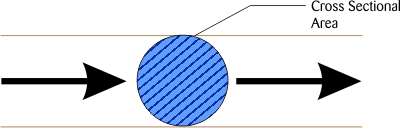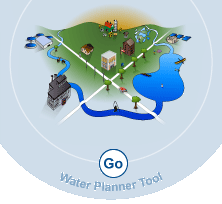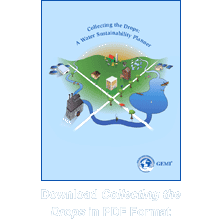Home Introduction Module 1: Water Use and Impacts Module 2: Water Management Risk Assessment Module 3: Case Examples and Links Calculators Reference# Fluid Flow Fundamentals

Water systems involve the movement of water from one point to another. Examples are transferring water from one process to another, or from one production facility or building to a packaging plant or final product shipping area.

Water usage is typically expressed as a volumetric flow rate (volume/time). If the water flowing though a cylindrical pipe is represented by the shaded area shown below (perpendicular to flow direction) and the velocity of the water is known, then the flow rate of the water can be determined through the pipe as gal/min or m3/sec.Flow Rate Calculator Velocity = ft/sec Cross Sectional Area = ft² Flow Rate = gpm SI Calculator Velocity = m/sec Cross Sectional Area = m² Flow Rate = L/S

## Flow Rate Equations

Flow Rate (Q) = Area x Velocity

The flow rates of process pipes are very important to know to complete water balances and usage and measuring volumetric flow rates are easy using common flow meters. See Types of Flow Meters for various types of flow measurement devices.

Sample Calculation: Determine the quantity of water flowing through a 6-inch diameter pipe that has a velocity of 5 ft/sec.

 Flow rate (cfs) = Velocity (ft/sec) x Pipe Cross Sectional Area (ft2) Flow rate (cfs) = 5 ft/sec x .196 ft2 Flow rate (cfs) = 0.98 ft3 sec Multiply 0.98 ft3 By 449 to get gpm sec Flow (gpm) = 0.98 x 449 = 440 gpm

## SI Units

 Flow M3 / S = Velocity (m/s) x Pipe Cross Sectional Area (m2) Flow M3 / S = 1.52 m/s x .018 m2 = .028 m3/sec Multiply 0.028 m3 /sec by 1000 to get L / S Flow L / S = 0.028 x 1000 Flow L / S = 28 L / S## Legend

 L/S = Liters per Second gpm = Gallons per Minute HP = Horsepower KW = Kilowatt m/s = Meters per Second m³ = Cubic Meters m² = Square Meters ft² = Square Feet ft³ = Cubic FeetGEMI
1155 Fifteenth Street, NW,
Suite 500
Washington, DC 20005
Phone: 202-296-7449
Fax: 202-296-7442
info@gemi.org